Drop a Query

# Probability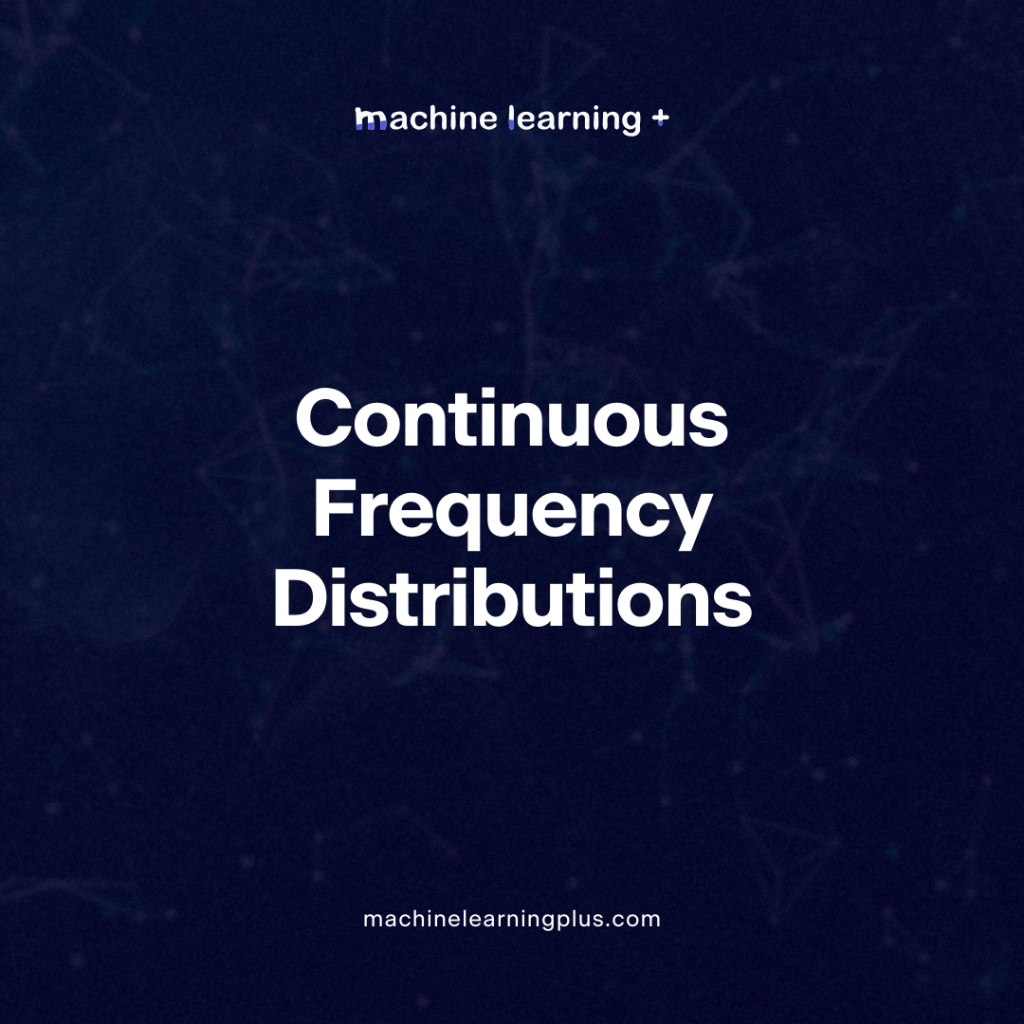## Continuous Frequency Distributions – Understanding Continuous Frequency Distributions and the Probability Density Function (PDF)

Lets deep dive into the world of statistics to understand the mysteries of continuous frequency distributions and the probability density function (PDF). By the end of this post, you’ll have a clearer understanding of these foundational concepts and their significance in the statistics. In this Blog post we will learn: Continuous Frequency Distributions: Constructing the …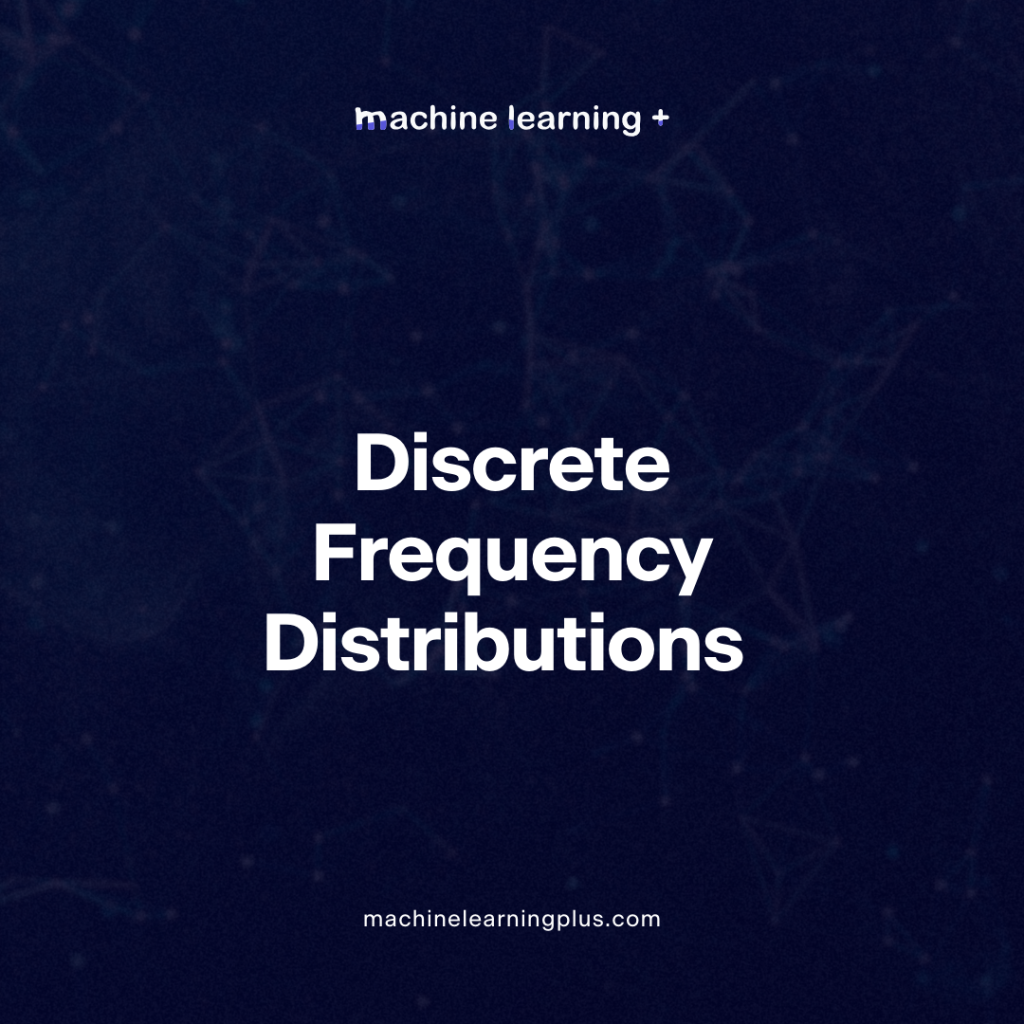## Discrete Frequency Distributions – Decoding Discrete Frequency Distributions and Probability Mass Functions (PMFs) with Real-World Scenarios

Probability and statistics are often viewed as complex areas of study. However, by using real-world examples and some visual aids, the seemingly challenging concepts become quite intuitive. Let’s deep dive into discrete frequency distributions and the different types of Probability Mass Functions (PMFs). In this Blog post we will learn: Discrete Frequency Distribution: Types of …

## Probability frequency distribution – A Comprehensive guide on probability frequency distribution with Examples

A probability frequency distribution is a way to describe the likelihood of an outcome. It gives a clear picture of how often an event can happen relative to all possible outcomes. Whether you’re studying data science, statistics, or merely interested in probability, understanding frequency distribution is crucial. In this Blog post we will learn: Types …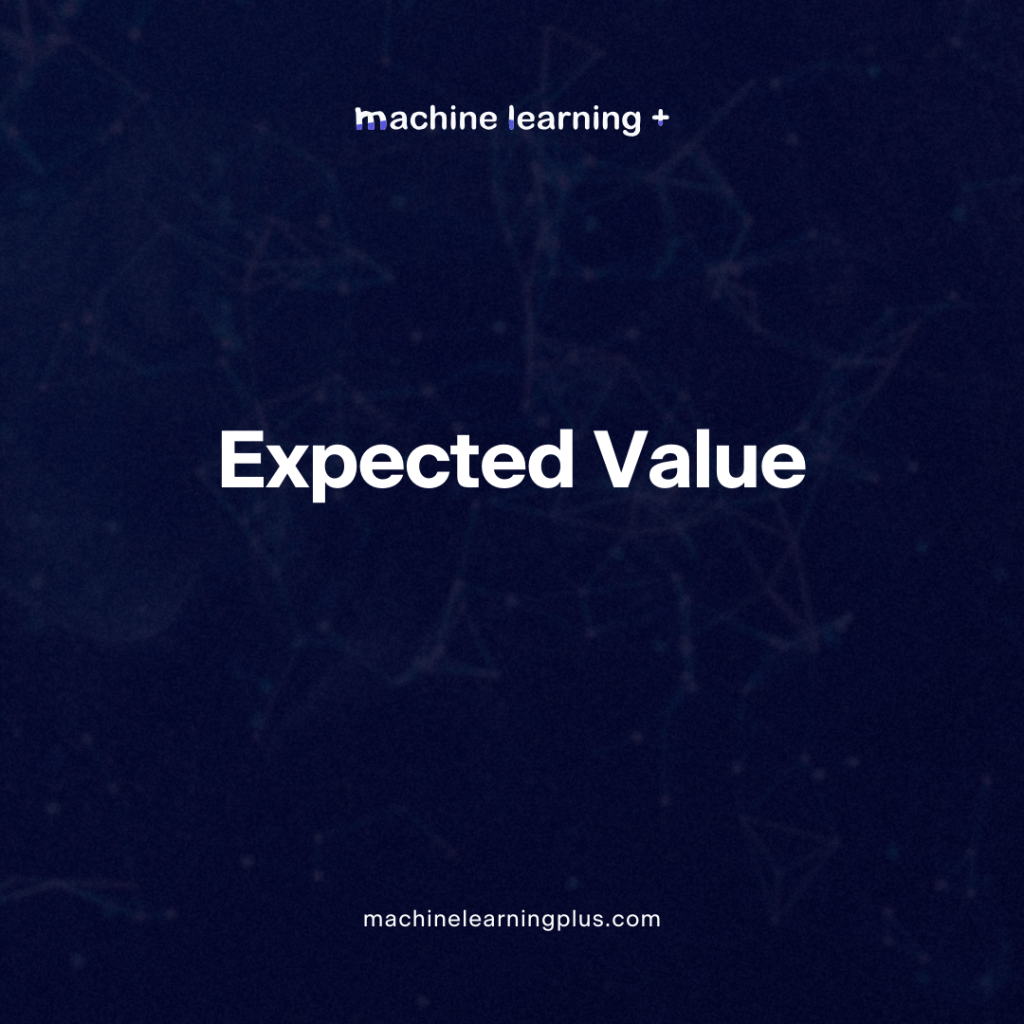## Expected Value – Understanding Expected Value in Probability and Its Real-Time Applications in Machine Learning

In the world of probability and statistics, the concept of the expected value plays a pivotal role. Whether we realize it or not, we make decisions based on expected outcomes in our daily lives. But what does this term mean mathematically, and how is it employed in machine learning? In this Blog post we will …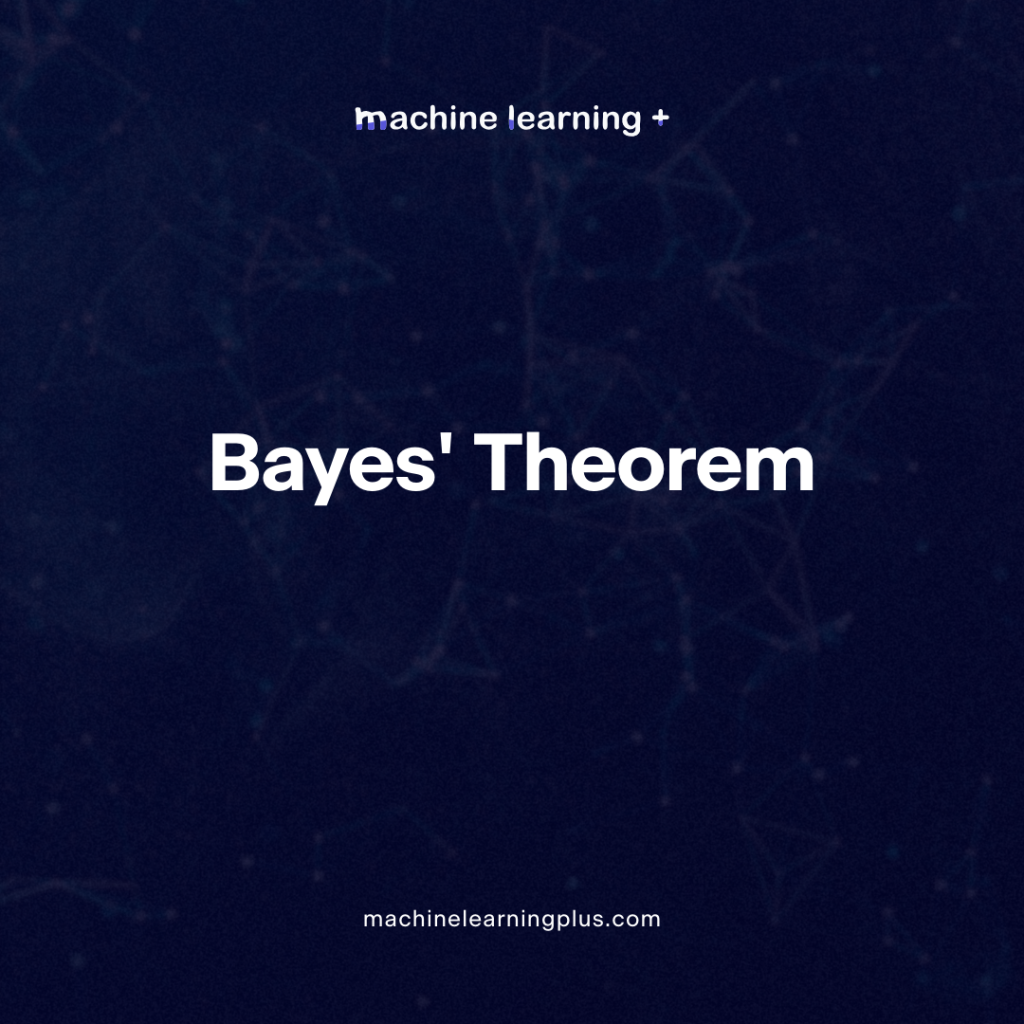## Bayes’ Theorem – Bayes’ Theorem and Bayesian Inference Unraveling the Mysteries of Probability

Understanding probability can be comparable to navigating a maze. Thankfully, Bayes’ theorem acts as our compass, particularly when we update our predictions with new information. Central to this theorem are three pivotal concepts: the prior, likelihood, and posterior. These elements pave the way for Bayesian inference, where Bayes’ theorem is used to renew the probability …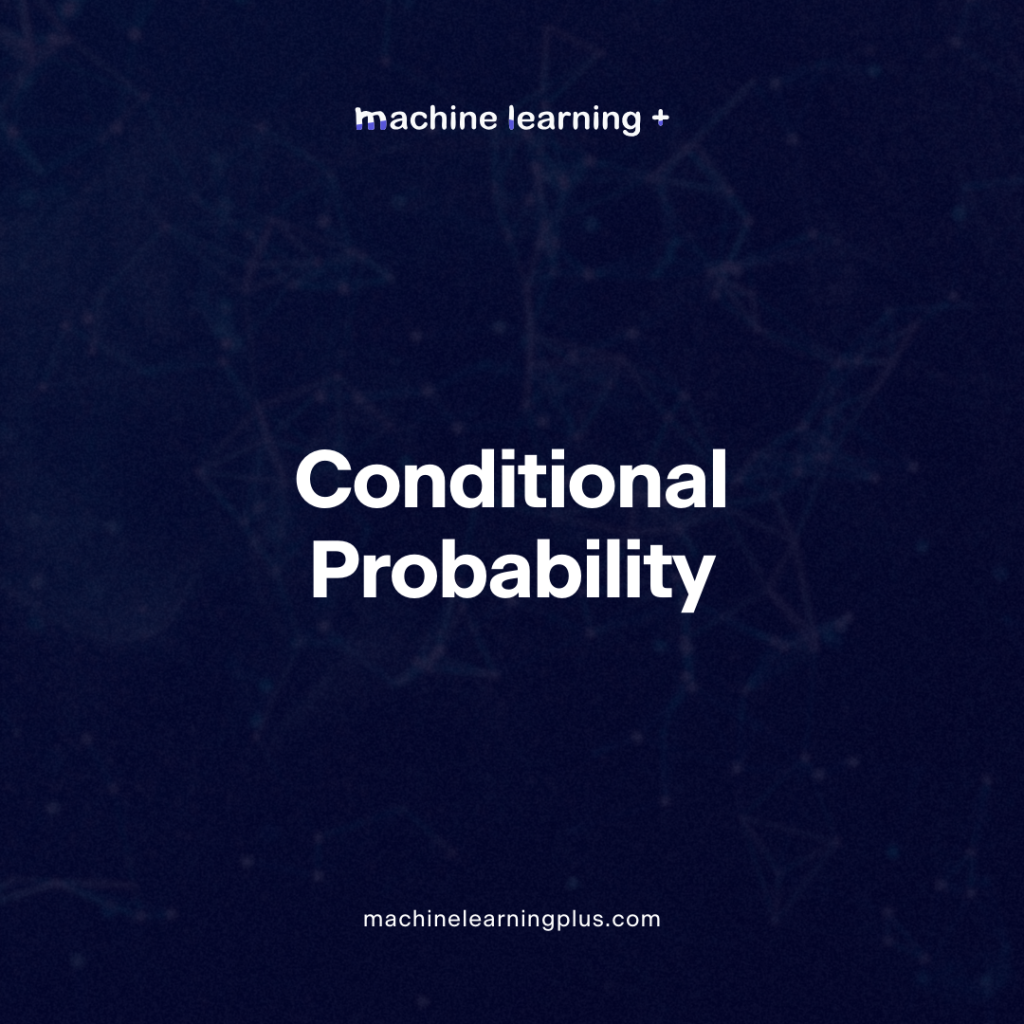## Conditional Probability – Understanding Conditional Probability with Examples

Probability is a field of study that deals with the likelihood of events occurring. One of the fundamental concepts in this field is “conditional probability.” At its core, conditional probability helps us understand the probability of an event occurring given that another event has already occurred. This concept is pivotal in many real-world scenarios, from …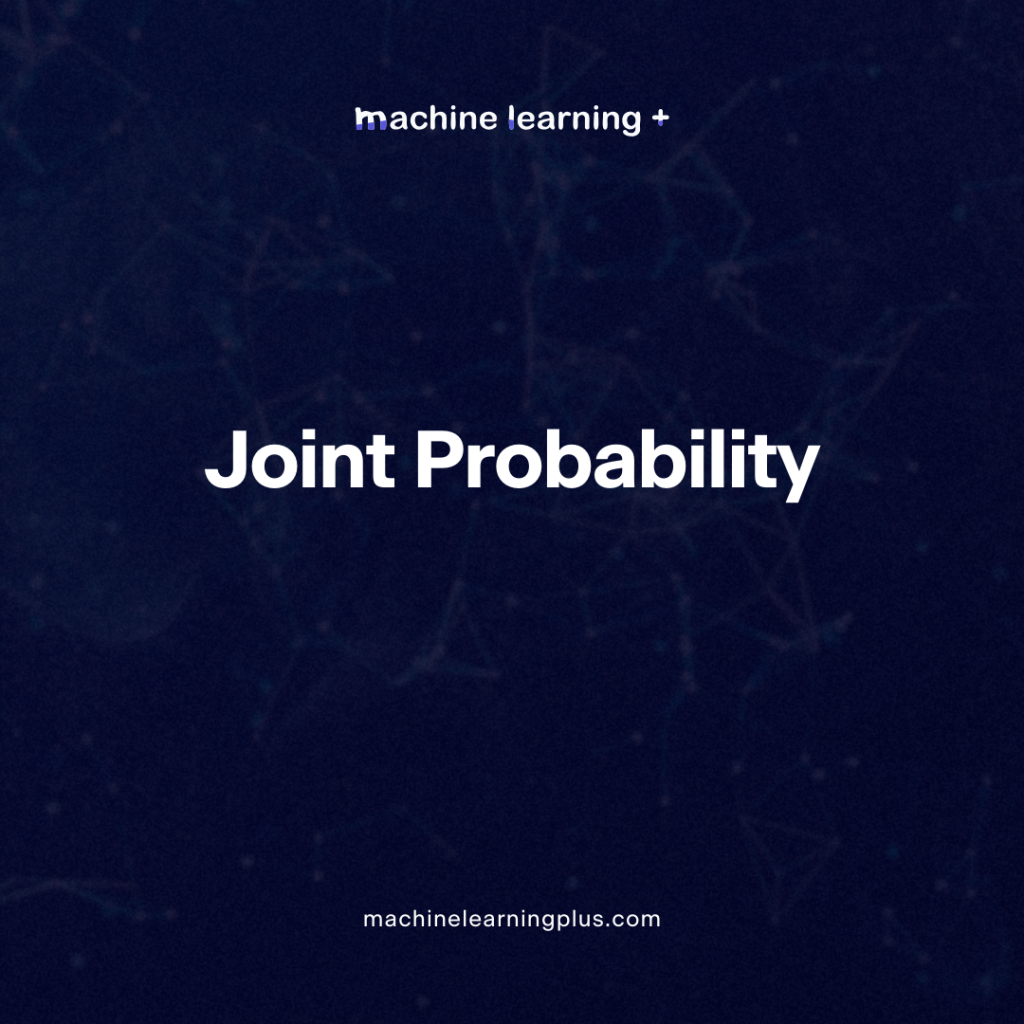## Joint Probability – A Comprehensive Guide with Examples

When dealing in probability, we encounter various concepts that provide a framework for understanding the uncertainty of events. One such foundational pillar is the joint probability. This measure informs us about the likelihood of two or more events occurring together. In this Blog post we will learn: What is Joint Probability? 1.1. Joint Probability Formula …## Mutually Exclusive Events – Understanding Mutually Exclusive Events in Probability with Examples

Probability, at its core, serves as a measure of the likelihood of an event’s occurrence. But when dealing with multiple events, how do they relate to one another? This brings us to the topic of “mutually exclusive events”. In this Blog Post we will learn: What are Mutually Exclusive Events? Real-Life Examples Mutually Exclusive vs. …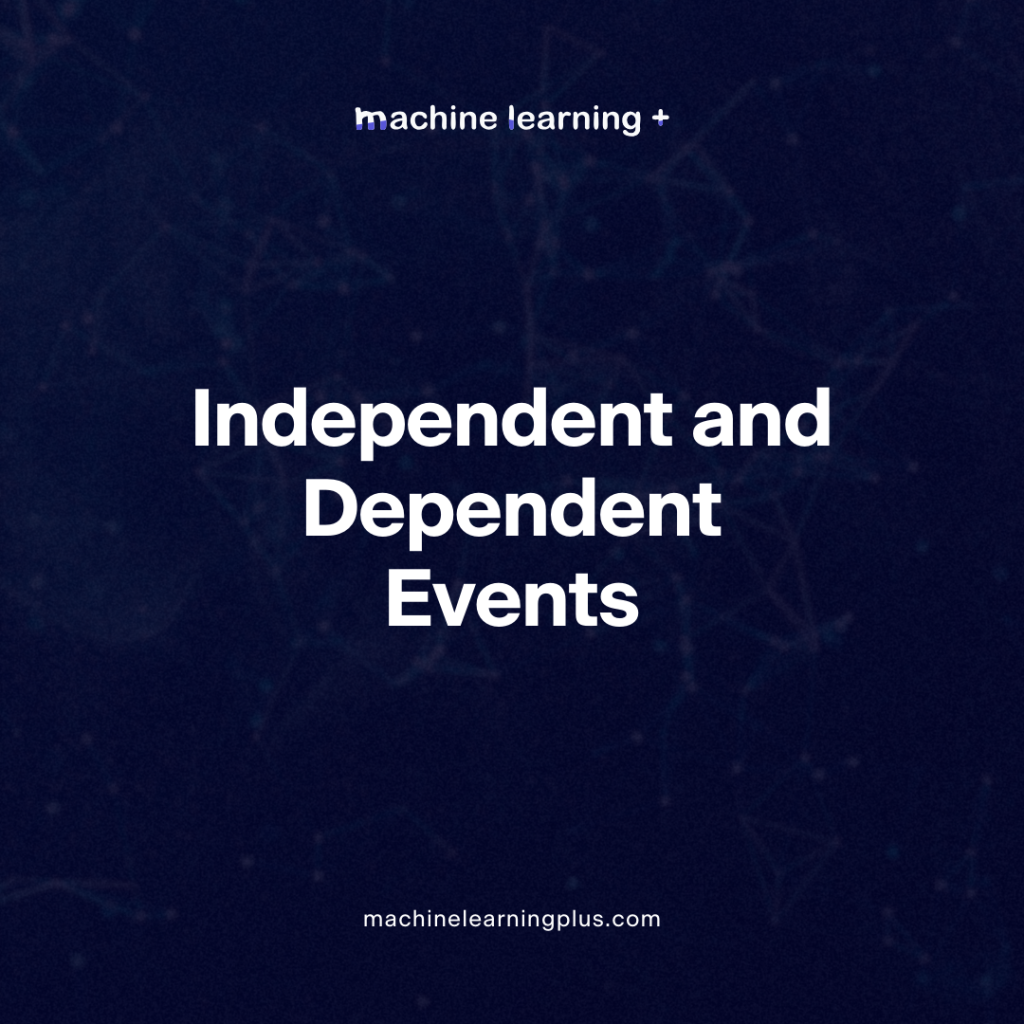## Independent and Dependent Events – Understanding Independent and Dependent Events in Probability

Probability, a fascinating branch of mathematics, helps us understand the likelihood of an event occurring. Within probability the concepts of independent and dependent events are pivotal. These two notions are key in defining and comprehending the interrelationships between various events. In this Blog Post we will learn: 1. Independent Events 2. Dependent Events 3. Key …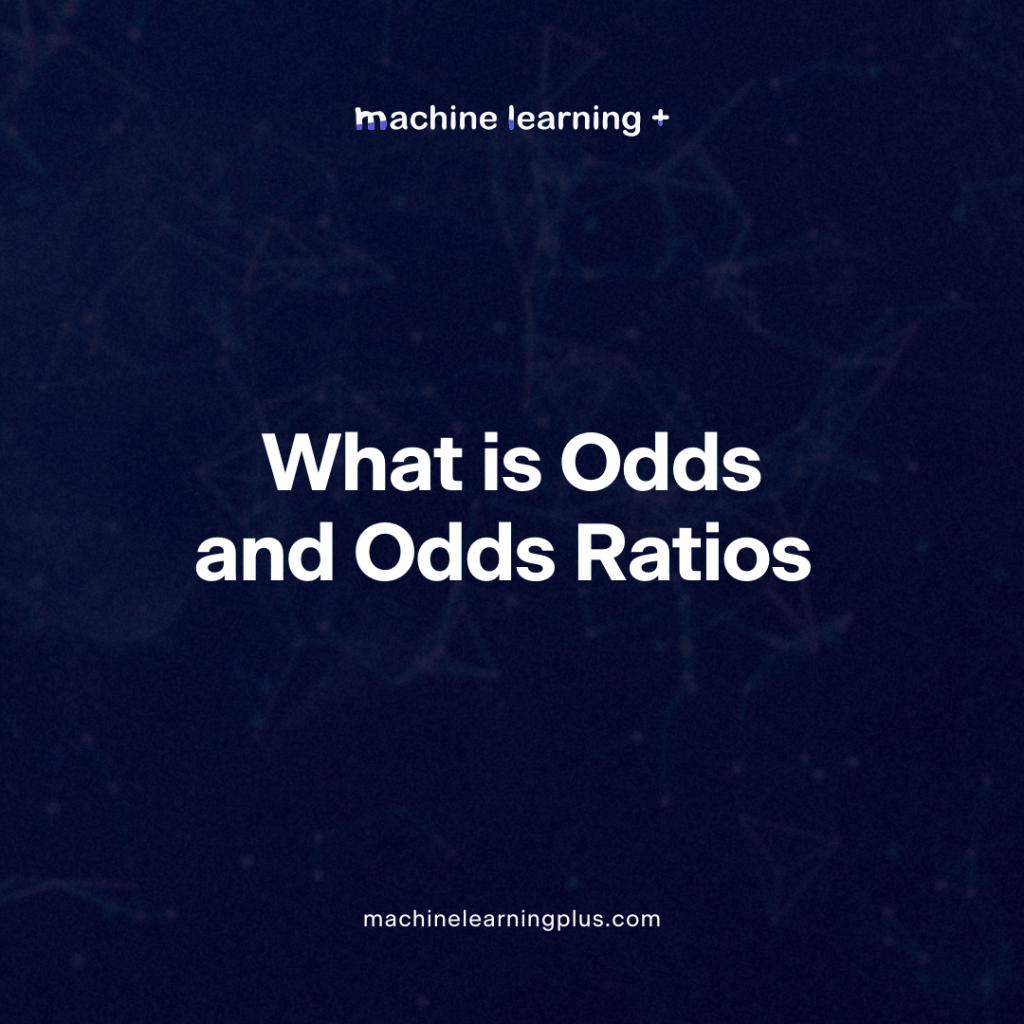## Odds and Odds Ratios – Understanding Odds and Odds Ratios in the World of Data Science

Probability, as a concept, plays an instrumental role in the world of data science. When we talk about probability, we’re essentially talking about quantifying the uncertainty or the chance of an event occurring. One term that often finds its way in probability is ‘odds’. Odds can be somewhat counterintuitive, especially for those who are familiar …Course Preview

## Machine Learning A-Z™: Hands-On Python & R In Data Science

### Free Sample Videos:#### Machine Learning A-Z™: Hands-On Python & R In Data Science#### Machine Learning A-Z™: Hands-On Python & R In Data Science#### Machine Learning A-Z™: Hands-On Python & R In Data Science#### Machine Learning A-Z™: Hands-On Python & R In Data Science